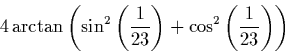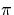Next: Exact Real Arithmetic Up: Approaches to Real Arithmetic Previous: Stochastic Rounding

## Symbolic Approaches

Using a symbolic approach to real arithmetic involves manipulating expressions in terms of symbols representing variables, constants, and functions, rather than performing computations with the numbers themselves.

Whilst an expression is manipulated solely in terms of its symbols, it represents exactly the correct result. For example, integration performed using floating point arithmetic will only represent an approximation to the correct result, but if the integration can be performed symbolically, the resulting expression will represent the answer exactly.

Symbolic approaches to arithmetic are extremely useful for certain tasks because they can provide information about results that a numeric approach would not be able to give. For example, simplifying an expression symbolically may reveal some useful property not apparent from a numerical answer. ConsiderSimplification reveals that this is equal to. This is probably more useful than the result which would be achieved by floating point approximation. It is worth noting that there are many complicated symbolic expressions for which we can find no useful simplification.

Unfortunately, although the symbolic approach is extremely useful in certain applications, it is not general enough to replace other approaches. In particular, although an expression may represent the correct result, when it becomes necessary to evaluate the expression to get a numeric answer, an approach such as floating point or interval arithmetic will need to be used. The accuracy of the evaluated result will still suffer from the problems which apply to to the arithmetic operations used to compute it.

There is no reason in principle why exact real arithmetic techniques should not be used in conjunction with the symbolic approach.Next: Exact Real Arithmetic Up: Approaches to Real Arithmetic Previous: Stochastic Rounding
Martin Escardo
5/11/2000